ICSE Class 10 Study of Compounds C. Nitric Acid Solution New

## ICSE Class 10 Study of Compounds C. Nitric Acid Solution By Clarify knowledge

ICSE Class 10 Study of Compounds C. Nitric Acid Solution New Pattern

## Best Reference Books For Class 10 ICSE

Best Reference Books For Class 10 ICSE Physics Is OSWAL GURUKUL

## Chapter 10 - Study of Compounds C. Nitric Acid Exercise Intext 1

Question 1

What is: (a) aqua fortis (b) aqua regia (c) Fixation of Nitrogen?Solution 1

(a) Aqua fortis: Nitric acid is called aqua fortis. Aqua fortis means strong water. It is so called because it reacts with nearly all metals.

(b) Aqua Regia: Conc. Nitric acid (1part by volume) when mixed with conc. Hydrochloric acid (3 parts by volume) gives a mixture called aqua regia. It means Royal water.

HNO3 +3HCl NOCl +2H2O +2[Cl]

(c) Fixation of Nitrogen: The conversion of free atmospheric nitrogen into useful nitrogenous compounds in the soil is known as fixation of atmospheric nitrogen.Question 2

During thunderstorm, rain water contains nitric acid. Explain with reactions.Solution 2

During lightning discharge, the nitrogen present in the atmosphere reacts with oxygen to form nitric oxide.

N2+ O2NO

Nitric oxide is further oxidized to nitrogen dioxide.

2NO +O22NO2

The nitrogen dioxide dissolves in atmospheric moisture in the presence of oxygen of the air and forms nitric acid which is washed down by the rain and combines with the salt present on the surface of the earth.

4NO2+2H2O +O2 4HNO3Question 3

Ammonia is used in the Ostwald process.

(a) Give the source of reactants used in the process.

(b) Name the catalyst used in the process.

(c) Name the oxidising agent used in this process.

(d) What is the ratio of ammonia and air taken in this process?

(e) Why is quartz used in the process?Solution 3

(a) A mixture of air and dry ammonia in the ratio of 10:1 by volume

(b) Platinum gauze

(c) Oxygen

(d) ammonia : dry air :: 1 : 10

(e) Quartz is acid resistant. When packed in layers, it helps in dissolving nitrogen dioxide uniformly in water.Question 4

(a) Write a balanced chemical equation for the laboratory preparation of nitric acid.

(b) In the preparation of nitric acid from KNO3, concentrated hydrochloric acid is not used in place of concentrated sulphuric acid. Explain why?

(c) Conc. Nitric acid prepared in the laboratory is yellow in colour .Why? How is this colour removed?

(d) Give reasons for the following:

In the laboratory preparation of nitric acid, the mixture of concentrated sulphuric acid and sodium nitrate should not be heated very strongly above 200oC.Solution 4

(a)Chemical equation is:

KNO+H2SO4 KHSO4 +HNO3

(b) Concentrated hydrochloric acid cannot replace Conc. Sulphuric acid for the preparation of nitric acid because hydrochloric acid is volatile acid and hence nitric acid vapours will carry HCl vapours.

(c) Conc. Nitric acid prepared in the laboratory is yellow in colour due to the dissolution of reddish brown coloured nitrogen dioxide gas in acid. This gas is produced due to the thermal dissociation of a portion of nitric acid.

4HNO3 2H2O + 4NO2 + O2

The yellow colour of the acid is removed:

If dry air or CO2 is bubbled through the yellow acid, the acid turns colourless because it drives out NO2 from warm acid which is further oxidized to nitric acid.

By addition of excess of water, nitrogen dioxide gas dissolves in water and thus the yellow colour of the acid is removed.

(d)The temperature of the mixture of concentrated sulphuric acid and sodium nitrate should not exceed 200oC because sodium sulphate formed at higher temperature forms a hard crust which sticks to the walls of the retort and is difficult to remove. At higher temperature nitric acid may also decompose.

NaNO3 + NaHSO4 Na2SO4 + HNO3Question 5(a)

Nitric acid cannot be concentrated beyond 68% by the distillation of dilute solution of HNO3. State the reason.Solution 5(a)

Nitric acid forms a constant boiling mixture with water containing 68% acid. This mixture boils constantly at constant boiling point without any change in its composition. At this temperature, the gas and the water vapour escape together. Hence the composition of the solution remains unchanged. So nitric acid cannot be concentrated beyond 68% by distillation of dilute solution of HNO3.Question 5(b)

What is passive iron? How is passivity removed?Solution 5(b)

Iron becomes inert when reacted with nitric acid due to the formation of extremely thin layer of insoluble metallic oxide which stops the reaction.

Passivity can be removed by rubbing the surface layer with the sand paper or by treating with strong reducing agent.Question 6

Name the product formed when :

(a) Carbon and conc. Nitric acid is heated

(b) Dilute HNO3 is added to copper.Solution 6

(a) When carbon and conc. Nitric acid is heated the products formed are Carbon dioxide, Nitrogen dioxide and water.

C + 4HNO3 CO2 + 2H2O +4NO2

(b) Copper when reacts with dilute HNO3 forms Copper nitrate, Nitric oxide and water.

3Cu + 8 HNO3 3Cu(NO32 +4H2O + 2NOQuestion 7

Give two chemical equations for each of the following:

(a) Reactions of nitric acid with non-metals.

(b) Nitric acid showing acidic character.

(c) Nitric acid acting as oxidizing agent.Solution 7

(a) Reaction of nitric acid with non-metals:

C + 4HNO3 CO2 + 2H2O + 4 NO2

S + 6 HNO3 H2SO4 + 2H2O + 6 NO2

(b) Nitric acid showing acidic character:

K2O + 2HNO3 2KNO3 + H2O

ZnO + 2HNO3 Zn(NO3)2 + H2O

(c) Nitric acid acting as oxidizing agent

P4 +20HNO3 4H3PO4 + 4H2O + 20NO2

3Zn +8HNO3 3Zn(NO3)2 +4H2O +2NOQuestion 8

Write the balanced equations and name the products formed when :

(a) Sodium hydrogen carbonate is added to nitric acid.

(b) Cupric oxide reacts with dilute nitric acid.

(c) Zinc reacts with dilute nitric acid.

(d) Concentrated nitric acid is heated.Solution 8

(a) When Sodium hydrogen carbonate is added to nitric acid sodium nitrate, carbon dioxide and water is formed.

NaHCO3 + HNO3 NaNO+H2O +CO2

(b) When Cupric oxide reacts with dilute nitric acid, it forms Copper nitrate.

CuO +2HNO3 Cu(NO3)2 +H2O

(c) Zinc reacts with nitric acid to form Zinc nitrate, nitric oxide and water.

3 Zn +8HNO3 3Zn(NO3)2 +4H2O +2NO

(d) 4HNO32H2O + 4NO2 + O2Question 9

How will you prepare the following from nitric acid?

(a) Sodium nitrate

(b) Copper nitrate

(d) Magnesium nitrate

(e) Ferric nitrate

(f) Aqua regiaSolution 9

(a) Sodium nitrate:

NaOH + HNO3 NaNO3 +H2O

Sodium hydroxide reacts with nitric acid to form sodium nitrate.

(b) Copper nitrate:

CuO + 2HNO3 Cu(NO3)2 + H2O

Copper oxide reacts with nitric acid to form copper nitrate.

Pb +4HNO3 Pb(NO3)2 +2H2O +2NO2

(d) Magnesium nitrate:

Mg +2HNO3 Mg(NO3)2 +H2

Magnesium with dil. nitric acid to form magnesium nitrate.

(e) Ferric nitrate:

Fe + 6HNO3 Fe(NO3)3 +3H2O + 3NO2

Iron reacts with conc. nitric acid to form ferric nitrate.

(f) Aqua regia:

HNO3 + 3HCl NOCl +2H2O +2[Cl]

Nitric acid reacts with hydrochloric acid to form a mixture called aqua regia.Question 10

Write the equation for following conversions A, B , C and D.

Solution 10

A: Copper can be converted into copper nitrate.

3Cu + 8HNO3Cu(NO3)2 + 4H2O+ 2NO

B:2Cu(NO3)2 2CuO + 4NO2 + O2

C:2Cu+ O2 2CuO

D:By reduction

2CuO + C 2Cu + CO2Question 11

Correct the following , if required:

(a) HNOis a strong reducing agent.

(b) NaNO3 gives NO2 and O2 on heating.

(c) Constant boiling nitric acid contains 80% nitric acid by weight.

(d) Nitric acid remains colourless even when exposed to light.Solution 11

(a) HNO3 is strong oxidizing agent.

(b) NaNO3 gives NaNO2 and oxygen on heating.

(c) Constant boiling nitric acid contains 68% nitric acid by weight.

(d) Nitric acid turns yellow solution when exposed to light.

## Chapter 10 - Study of Compounds C. Nitric Acid Exercise Ex. 10

Question 1

(a) The nitrate salt which does not give a mixture of NO2 and O2 on heating is:

(i) AgNO3

(ii) KNO3

(iii) Cu(NO3)2

(iv) Zn(NO3)2

(b) The chemical used in the brown ring test is:

(i) CuSO4

(ii) FeSO4

(iii) Fe2(SO4)3

(iv) ZnSO4

(c) Lead nitrate decomposes on heating to give:

(i) NO

(ii) N2O

(iii) NO2

(iv) N2O5Solution 1

(a)KNO3

(b) FeSO4

(c) NO2Question 2

Name:

(a) A nitrate of metal which on heating does not give nitrogen dioxide.

(b) A nitrate which on heating leaves no residue behind.

(c) A metal nitrate which on heating is changed into metal oxide.

(d) A metal nitrate which on heating is changed into metal.

(e) A solution which absorbs nitric oxide.

(f) The oxide of nitrogen which turns brown on exposure to air. How is it prepared?Solution 2

(a) Sodium nitrate

2NaNO3 2NaNO2 +O2

(b) A nitrate which on heating leaves no residue behind- Ammonium nitrate.

(c) A metal nitrate which on heating is changed into metal oxide- Calcium nitrate

(d) A metal nitrate which on heating is changed into metal- Silver nitrate

(e) A solution which absorbs nitric oxide- Freshly prepared ferrous sulphate

(f) The oxide of nitrogen which turns brown on exposure to air. - nitric oxide

By catalytic oxidation of ammonia.

4 NH3 + 5 O2 4 NO + 6 H2O + HeatQuestion 3

Mention three important uses of nitric acid. Give the property of nitric acid involved in the use.Solution 3

Three important uses of Nitric acid and the property of nitric acid involved is:

Question 4

(a) Explain with the help of a balanced equation, the brown ring test for nitric acid.

(b) Why is freshly prepared ferrous sulphate solution used for testing the nitrate radical in the brown ring test?Solution 4

(a) Brown ring test

Procedure:

(i) Add freshly prepared saturated solution of iron (II)sulphate to the aq. solution of nitric acid.

(ii) Now add conc. Sulphuric acid carefully from the sides of the test tube, so that it should not fall drop wise in the test tube.

(iii) Cool the test tube in water.

(iv) A brown ring appears at the junction of the two liquids.

(b)A freshly prepared ferrous sulphate solution is used because on exposure to the atmosphere, it is oxidized to ferric sulphate which will not give the brown ring.Question 5

From the following list of substances, choose one substance in each case which matches the description given below:

Ammonium nitrate, Calcium hydrogen carbonate, copper carbonate, lead nitrate, potassium nitrate, sodium carbonate, sodium hydrogen carbonate, zinc carbonate.

(a) A nitrate which gives off only oxygen when heated.

(b) A nitrate which on heating decomposes into dinitrogen oxide (nitrous oxide) and steam.

(c) A nitrate which gives off oxygen and nitrogen dioxide when heated.

(d) A substance which on heating leaves yellow residue.Solution 5

(a) Potassium nitrate

(b) Ammonium nitrate

The action of heat on the blue crystalline solid X gives a reddish brown gas Y, a gas which re-lights a glowing splint and leaves a black residue. When gas Z, which has a rotten egg smell, is passed through a solution of X, a black ppt. is formed.

a. Identify X, Y and Z.

b. Write the equation for action of heat on X.

c. Write the equation between solution X and gas Z.Solution 6

a. X is copper nitrate.

Y is nitrogen dioxide.

Z is hydrogen sulphate.

b.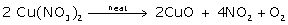c.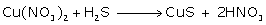Question 7

X, Y and Z are three crystalline solids which are soluble in water and have common anion.

To help you to identify X, Y and Z you are provided with the following experimental observations. Copy and complete the corresponding inferences in (a) to (f).

(a) A reddish -brown gas is obtained when X, Y and Z are separately warmed with concentrated sulphuric acid and copper turning added to the mixture.

Inference 1: The common anion is the _____ion.

(b) When X is heated, it melts and gives off only one gas which re-lights a glowing splint.

Inference2: The cation in X is either _____ or _____.

(c) The action of heat on Y produces a reddish brown gas and yellow residue which fuses with glass of the test tube.

Inference3: The metal ion present in Y is the____ ion.

(d) When Z is heated, it leaves no residue. Warming Z with sodium hydroxide solution liberates a gas which turns moist red litmus paper blue.

Inference4: Z contains the ____ cation.

(e) Write the equations for the following reactions:

(1)X and concentrated sulphuric acid (below 200oC). (One equation only for either of the cations given in Inference 2)

(2)Action of heat on Y.

(3) Concentrated nitric acid is added to copper turnings kept in a beaker.Solution 7

(a) Nitrate.

(b) Sodium or potassium

(d) Ammonia

(e) (1)KNO3 + H2SO4 KHSO4 + HNO3

(2) 2Pb(NO3)2 2PbO + 4NO2 +O2

(3) Cu +4HNO3 Cu(NO3)2 +H2O +2NO2Question 8

a. Dilute nitric acid is generally considered a typical acid except for its reaction with metals. In what way is dilute nitric acid different from other acids when it reacts with metals?

b. Write the equation for the reaction of dilute nitric acid and conc. nitric acid with copper.Solution 8

a. Dilute nitric acid is generally considered a typical acid except for its reaction with metals because it does not liberate hydrogen. It is a powerful oxidising agent, and nascent oxygen formed oxidises hydrogen in water.

b.

i. Reaction of dilute nitric acid with copper:

3Cu + 8HNO3→ 3Cu(NO3) + 4H2O + 2NO

ii. Reaction of conc. nitric acid with copper:

Cu + 4HNO3→ Cu(NO3) + 2H2O + 2NO2Question 9

Explain why:

a. Only all-glass apparatus should be used for the preparation of nitric acid by heating concentrated sulphuric acid and potassium nitrate.

b. Nitric acid is kept in a reagent bottle for a long time.Solution 9

a. The glass apparatus is purposely used because nitric acid vapours are highly corrosive in nature and corrode cork, rubber etc. if used as a stopper.

b. Pure nitric acid is unstable to heat or sunlight. In the presence of sunlight, it decomposes even at room temperature.

Nitric acid stored in a bottle turns yellow. This colour is due to dissolved NO2 in HNO3. To avoid decomposition, nitric acid is normally stored in coloured bottles.Question 10

The figure given below illustrates the apparatus used in the laboratory preparation of nitric acid.

a. Name A (a liquid), B (a solid) and C (a liquid). (Do not give the formulae).

b. Write an equation to show how nitric acid undergoes decomposition.

c. Write the equation for the reaction in which copper is oxidised by concentrated nitric acid.Solution 10

a. A (a liquid): Conc.  sulphuric acid

B (a solid): Sodium nitrate

C (a liquid): Nitric acid

b.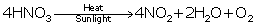c.

i. Reaction of dilute nitric acid with copper:

3Cu + 8HNO3→ 3Cu(NO3) + 4H2O + 2NO

ii. Reaction of conc. nitric acid with copper:

Cu + 4HNO3→ Cu(NO3) + 2H2O + 2NO2Question 11

a. A dilute acid B does not normally give hydrogen when reacted with metals but does give a gas when reacts with copper. Identify B. Write the equation with copper.

b. Complete the table:

c. What is the property of nitric acid which allows it to react with copper?

d. State one observation:

(i) Concentrated nitric acid is reacted with sulphur.
(ii) Lead nitrate is heated strongly in a test tube.Solution 11

a. The dilute acid is nitric acid.

Reaction of dilute nitric acid with copper:

3Cu + 8HNO→3Cu(NO3) + 4H2O + 2NO

b.

c. Its oxidising property allows it to react with copper.

d.
(i) Reddish brown gas of NO2 is observed.
(ii) Yellow solid is formed which fuses with glass.Question 2012

a. Name the gas produced when copper reacts with conc. HNO3.

b. State your observation: Zinc nitrate crystals are strongly heated.

c. Correct the statement: Magnesium reacts with nitric acid to liberate hydrogen gas.

d. Iron is rendered passive with fuming HNO3. Give reason.

e. Give the balanced equation for dilute nitric acid and copper carbonate.Solution 2012

a. Nitrogen dioxide gas is produced when copper reacts with conc. HNO3.

b. When zinc nitrate crystals are strongly heated, they decompose into yellow-coloured zinc oxides and nitrogen dioxides, and oxygen gas is liberated.

c. Very dilute (about 1%) acid reacts with magnesium at room temperature to give magnesium nitrate and hydrogen gas.

d. Iron is rendered passive with fuming HNO3. This is due to the formation of insoluble metallic oxide which stops the reaction.

e.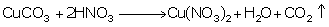Question 2013

a. Identify the gas evolved when

i. Sulphur is treated with conc. nitric acid.

ii. A few crystals of KNO3 are heated in a hard glass test tube.

b. State two relevant observations for: Lead nitrate crystals are heated in a hard glass test tube.

c. Give a balanced equation for: Oxidation of carbon with conc. HNO3.Solution 2013

a.

i. When sulphur is treated with conc. nitric acid, it produces nitrogen dioxide gas.

ii. When a few crystals of KNO3 are heated in a hard glass test tube, it decomposes to form KNO2, and Ogas is librated.

b. First, it decomposes with slight decrepitation, and second, it is reddish brown in colour when hot. After cooling, it turns yellow and fuses in glass.

c.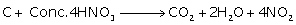Question 2014

a. Fill in the blank:

Cold dil. nitric acid reacts with copper to form (hydrogen, nitrogen dioxide, nitric oxide).

b. Give balanced equations for the following:

i. Laboratory preparation of nitric acid.

ii. Action of heat on a mixture of copper and nitric acid.Solution 2014

a. Nitric oxide

b.

i.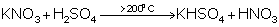ii. Reaction of dilute nitric acid with copper:

3Cu + 8HNO3→ 3Cu(NO3) + 4H2O + 2NO Reaction of conc. nitric acid with copper:

Cu + 4HNO3→ Cu(NO3) + 2H2O + 2NO2Question 2015 (a)

(a) Identify the acid

(i) The acid which is used in the preparation of a non-volatile acid.

(ii) The acid which is prepared by catalytic oxidation of ammonia. Solution 2015 (a)

(i) Sulphuric acid

(ii) Nitric acid Question 2015 (b)

State one relevant observation for each of the following:

When crystals of copper nitrate are heated in a test tube. Solution 2015 (b)

Reddish brown nitrogen dioxide gas is released, and the residue left behind is black copper oxide. Question 2015 (c)

Explain the following:

(i) Dilute nitric acid is generally considered a typical acid but not so in its reaction with metals.

(ii) Concentrated nitric add appears yellow when it is left standing in a glass bottle.

(iii) An all glass apparatus is used in the laboratory preparation of nitric acid.Solution 2015 (c)

(i) Dilute nitric acid is generally considered a typical acid but not in its reaction with metals, because the action of nitric acid on metals depends on the temperature and concentration of nitric acid. These conditions are not required in case of hydrochloric acid or sulphuric acid.

(ii) Although pure concentrated nitric acid is colourless, it appears yellow when left standing in a glass bottle due to the dissolution of reddish brown nitrogen dioxide gas in the acid. Nitrogen dioxide is produced because of the thermal decomposition of a portion of nitric acid.

4HNO3 → 2H2O + 4NO2 + O2

(iii) An all-glass apparatus is used in the laboratory preparation of nitric acid, because nitric acid vapour corrodes rubber and cork.

error: Content is protected !!